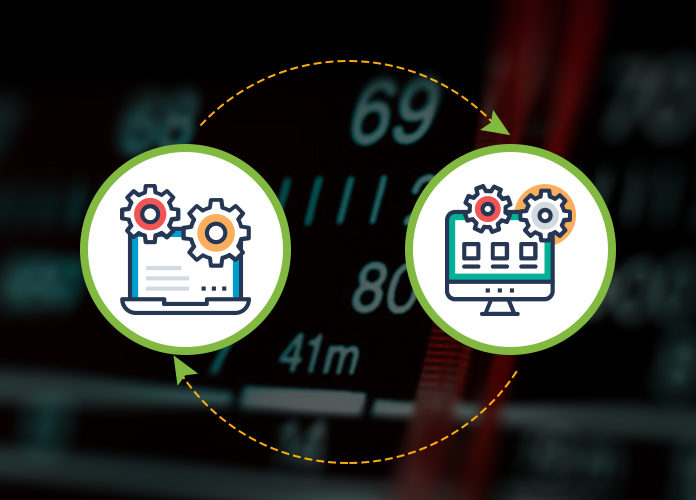# Hyperparameter Tuning In Neural Networks

0
1898In majority of the machine learning algorithms, the Hyperparameter are set before the model’s parameters are actually optimized. We can think of setting of the hyperparameters as to be selecting which model to use. It is like choosing the model that is to be used from a hypothesized set of appropriate models.

There can be many hyperparameters for a neural network. These hyperparameters can include the ones that determine how a neural network is trained, and also the ones that specify the structure of a the neural network itself.

## Some examples of hyperparameters for neural networks are as follows:

• Number of hidden units
• Learning rate
• Convolution kernel width

### Difference between a Parameter and a Hyperparameter

There are many jargons in machine learning, and it is very easy to get confused. “Parameter” and “Hyperparameter” are two such confusing terms. Let’s find out the difference between them.

A model parameter is basically a configuration variable whose value can be estimated from data. It is internal to a model. The parameters can be learnt from the historical training data. The parameters are generally not set manually, and are learnt by the model. The coefficients in a linear regression, the weights in a neural network, the support vectors in a support vector machine are some of the examples of model parameters.

A model hyperparameter, on the other hand, is a configuration that cannot be estimated from the data. It is external to a model. A hyperparameter can be set using heuristics. The learning rate for training a neural network, the k in k-nearest neighbours, the C and sigma in support vector machine are some of the examples of model hyperparameters.

At many places, the terms “parameter” and “hyperparameter” are used interchangeably, making things even more confusing. To avoid confusion, you should remember that if you need to specify some configuration variable manually, then it is not a model parameter, but is a model hyperparameter.

### Grid Search

Grid search is a very basic method for tuning hyperparameters of neural networks. In grid search, models are built for each possible combination of the provided values of hyperparameters. These models are then evaluated and the one that produces the best results is selected.

The grid search is inefficient because the entire hyperparameter space is sampled exhaustively, and each of the models is then trained using the training data and is evaluated using the test data.

### Random Search

Random search is a method of hyperparameter tuning, in which, a statistical distribution is provided for each hyperparameter, and the values of the hyperparameters are sampled randomly from their corresponding statistical distributions.

Random search works better than Grid search, especially if the performance of the model is affected by a few hyperparameters only. Also, using random search, you can specify different statistical distributions for different hyperparameters; which is a way by which you can utilize your prior knowledge about the hyperparameters.

### Bayesian Optimization

In the above two methods of finding suitable hyperparameters, many experiments are done before arriving at the appropriate set of hyperparameters. Those processes were parallel as the experiments could be held at same time and were independent of each other. Hence, the results of one experiment would be of no use in the other experiment. Sequential Model-Based Optimization (SMBO) algorithms allow the use of results of experiments in a previous iteration to help improve the hyperparameter selection for the further experiments in the next iteration. Bayesian Optimization is one such SMBO algorithm.

In order to reduce the number of iterations until a good configuration is found, adaptive Bayesian methods are invented. They select the next point for verification, taking into account the results at already checked points. The idea is to find at each step a compromise between:

• Exploring the regions near the most successful points among those found.
• Exploring regions with great uncertainty where even more successful points can be located.

This is often called the explore-exploit dilemma or “learning vs earning”. Thus, in situations where the verification of each new point is expensive (in machine learning, verification = learning + validation), one can approach the global optimum in a much smaller number of steps.

### Population Learning of Neural Networks

Population learning of neural networks, as well as random search, begins with parallel learning of the population of neural networks with random values ​​of hyperparameters. But, instead of training neural networks independently, from time to time, they are interrogated to refine the hyperparameters of models, based on the hyperparameters of those models that claim to be optimal. The population approach is inspired by genetic algorithms in which each member of the population receives information from other members and can, for example, copy the parameters of the most effective models or investigate the possibility of variation of their current values.

As the training progresses, the copying process and the variations of the best hyperparameters found by the population are periodically performed, so that all models in the population, at each time point, have a good basic level of productivity. At the same time, new hyperparameters are constantly being studied. Thus, population-based learning of neural networks makes it possible to optimize hyperparameters in the learning process, and to concentrate computing resources on hyperparameters and weight spaces for which it is most likely to obtain good learning outcomes. The result is a method for configuring hyperparameters, simultaneously leading to rapid learning and less demanding on computing resources.

It has also been found that population learning effectively works when learning a generative and adversarial network, the task of tuning of which remains a difficult problem. The approach was applied to modern Google machine translation systems. The selection of hyperparameters in machine delivery neuronets is usually done using the manual configuration method and takes months of optimization. Population training has allowed to exceed modern results without long repeated runs.